# Reasoning - Questions on Figure Series

### Score 0

1.Answer (a). Sum of the squares of the numbers in the first 2 circles is equal to the square of the number in the below circle. 3²+ 4²= 5² and 6²+ 8²= 10². Similarly 12²+ 5²= 13².

2.Answer (c). First figure 5²- 4²= 3², Second figure 13² - 5²= 12². Similarly 15² - 9²= 12².

3.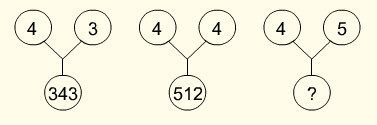Answer (a). 4+3=7, 7³=343. 4+4=8, 8³=512. Therefore 4+5=9, 9³=729

4.Answer (c). From first figure 6+5=11, 11x2=22. Second figure 8+7=15, 15x2=30. Similarly 12+11=23, 23x2=46.

5.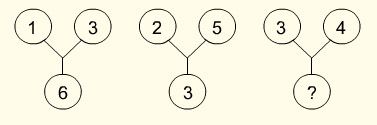Answer (a). Sum of the numbers in all the figures is equal to 10. Therefore the missing number in the third figure is 10 - 7 = 3.

6.Answer (d). All the numbers here are all prime. The next prime number in the series is 23.

7.Answer (a). First figure 4 + 5 = 9, √9 = 3. Second figure 7 + 9 = 16, √16 = 4. Third figure 7² = 49, 49 - 28 = 21.

8.Answer (d). First figure 9 - 5 = 4; 4 x 2 = 8. Second figure 17 - 11 = 6; 6 x 2 = 12. 19 - 12 = 7; 7 x 2 = 14.

9.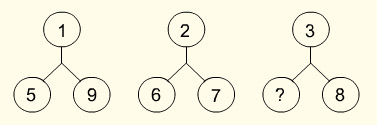Answer (b). Sum of all the numbers in the figures is 15. Therefore 8+3+x=15; x=15-11; x=4.

10.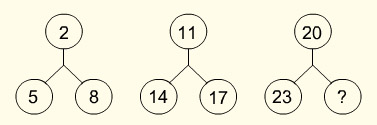Answer (a). The numbers increase by 3 from the first number 2. 2, 5, 8, 11, 14, 17, 20, 23, 26

11.Answer (d). First figure 19 - 17 = 2; 2 x 3 = 6, second figure 33 - 31 = 2, 2 x 4 = 8, therefore in the third figure 53 - 51 = 2, 2 x 5 = 10.

12.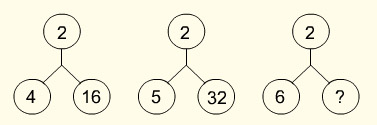Answer (c). First figure 24 = 16, Second figure 25 = 32, Third figure 26 = 64.

13.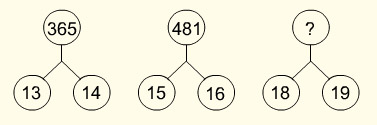Answer (a). First figure 13² + 14² = 365, second figure 15² + 16² = 481. Finally the third figure 18² + 19² = 685.

14.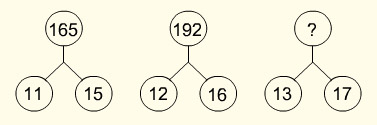Answer (c). The sequence in the figures is 11 x 15 = 165, 12 x 16 = 192 and 13 x 17 = 221.

15.Answer (d). The series is (52) ÷ 1 = 25, (62) ÷ 2 = 18, 102) ÷ 4 = 25.